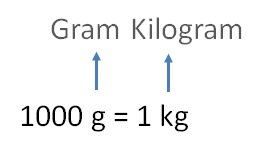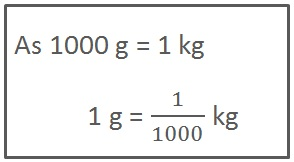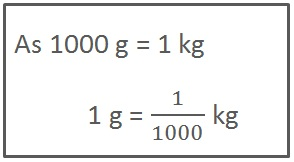Using Decimals - Converting to mm, cm, km, kg, rupees

Chapter 8 Class 6 Decimals
Concept wise

### Converting weight - g to kg1 g = 1/1000 kg

### Convert 28 g into kg

28 g  = 28 × 1 g

= 28 × 1/1000 kg= 28/1000 kg

= 0028/1000

= 0.028 kg

### Convert 8560 g into kg

8560 g  = 8560 × 1 g

= 8560 × 1/1000 kg

= 8560/1000 kg

= 8.560 kgLearn in your speed, with individual attention - Teachoo Maths 1-on-1 Class

### Transcript

Converting weight - g to kg1000 g = 1 kg 1 g = 1/1000 kg Convert 28 g into kg 28 g = 28 1 g = 28 1/1000 kg = 28/1000 kg = 0028/1000 = 0.028 kg Convert 8560 g into kg 8560 g = 8560 1 g = 8560 1/1000 kg = 8560/1000 kg = 8.560 kg